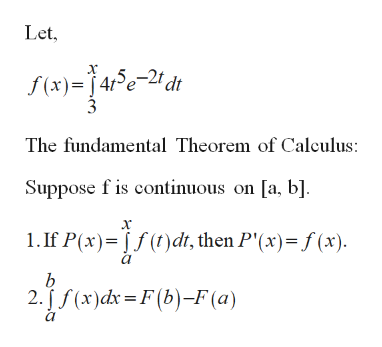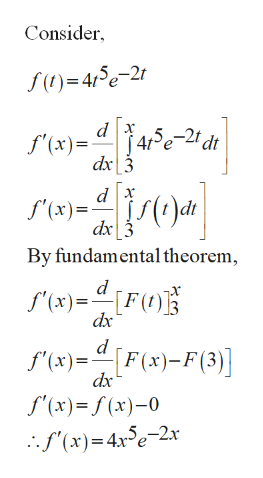# Can anyone plz explain how to do these.i really need help on this. Plz help.

Question
31 views

Can anyone plz explain how to do these.i really need help on this. Plz help.

check_circle

Step 1

Since you have asked multiple questions, we will solve the first question for you. If you want any specific question to be solved then please specify the question number or post only that question.

But the first question has multiple sub-parts, we will solve first three sub-parts for you. To get remaining sub-part solved please repost the complete question and mention the sub-parts to be solved.

Part (a):

Evaluate f’(x).help_outlineImage TranscriptioncloseLet, х 45e-21 dt f(x)4 The fundamental Theorem of Calculus Suppose fis continuous on [a, b] 1. If P(x) )dt, then P'(x)=f(x) b 2.f(x)dx=F(b)-F (a) fullscreen
Step 2

Differentiate the function f’(x).help_outlineImage TranscriptioncloseConsider )=45e-21 J4re-2t dt f'(x) dx 3 dx f'(x)= dx By fundamental theorem f'x)F() dx d f'(x) [F(x)-F(3) f'(x)f(x)-0 (x)4e-2x fullscreen
Step 3

Part (b):

Evaluate f’(...

### Want to see the full answer?

See Solution

#### Want to see this answer and more?

Solutions are written by subject experts who are available 24/7. Questions are typically answered within 1 hour.*

See Solution
*Response times may vary by subject and question.
Tagged in

### Other